# 分组与汇总

## 计算唯一值

 A 1 =file("Order_Books.txt").import@t() 2 =A1.id(SalesID) 3 =A1.id(PName)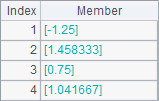A2中，取出所有不同的销售员编号SalesIDA3中取出所有不同的书籍名称PNameA2A3中的结果如下：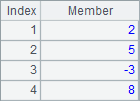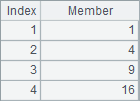A 1 =demo.query("select * from EMPLOYEE") 2 =A1.id(STATE) 3 =demo.query("select distinct STATE from EMPLOYEE")

A1中从数据库中选出了员工资料表如下：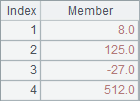A2中用id函数从A1的序表中选出所有不同的州，而A3中用distinct语句从数据库中获取结果。A2A3中的结果如下：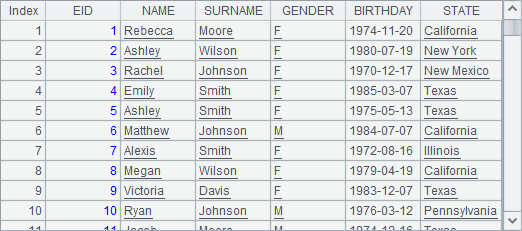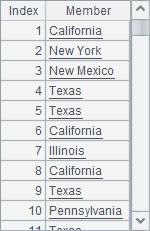A 1 =demo.query("select * from EMPLOYEE") 2 =A1.id@o(STATE)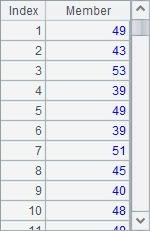A 1 =demo.query("select * from EMPLOYEE") 2 =A1.id@u(age(BIRTHDAY))

A2中列出了员工的年龄，这里使用了@u选项，结果中不会出现重复值，但会按照出现的先后顺序排列：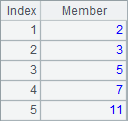## 等值分组

 A 1 =demo.query("select * from EMPLOYEE") 2 =A1.group(DEPT) 3 =A1.group@o(DEPT) 4 =A1.group(year(BIRTHDAY))

A2将员工按部门DEPT分组，结果如下：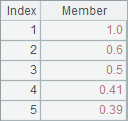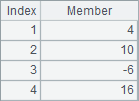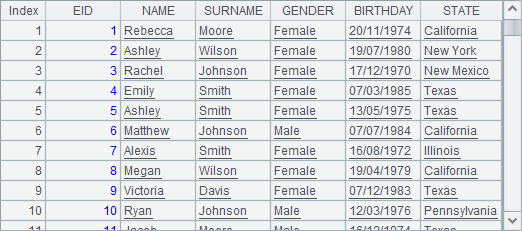group函数类似SQL中的group by运算。它的返回值是由各个分组构成的序列，最后结果是个由序列构成的序列。分组汇总值可基于分组结果继续多次计算。这与SQL不同，SQL中没有显式的集合数据类型，不能保存分组结果，必须在group by后立即计算出分组汇总值，运算后分组结果将被丢弃而不能重复利用。

 A 1 =demo.query("select * from EMPLOYEE") 2 =A1.group(DEPT) 3 =A2.new(DEPT,count(~):Count,~.sum(SALARY):TotalSalary) 4 =A2.new(DEPT,~.count(GENDER=="M"):Male, ~.count(GENDER=="F"):Female)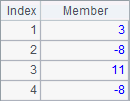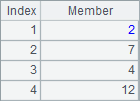SQL中的group by类似，group函数也支持同时对多个字段（表达式）分组，此时只有多个字段的值均相等才会分到同一组。

 A 1 =z(123,40) 2 =demo.query("select * from EMPLOYEE") 3 =A2.group(z(#,60)) 4 =A3.new(#:GID,~.count():Count,~:Emps)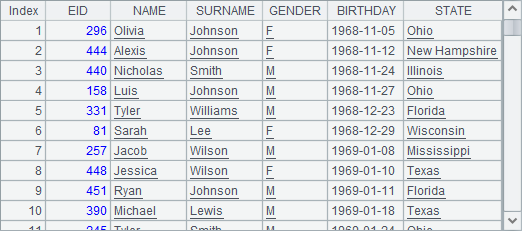A3中，则利用z函数，将员工数据按照每60个一组来分组，在A4中统计出了每一组的员工总数，计算后，A4中结果如下：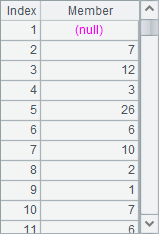A 1 =demo.query("select * from EMPLOYEE") 2 =A1.len() 3 =A1.group(z(#,6,A2)) 4 =A3.new(#:GID,~.count():Count,~:Emps)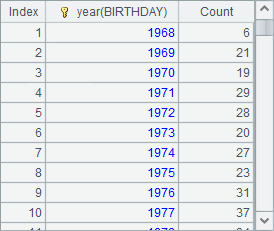## 分组汇总

 A 1 =demo.query("select * from EMPLOYEE") 2 =A1.group(DEPT;~.count():Count,~.sum(SALARY):TotalSalary) 3 =A1.group(DEPT;~.count(GENDER=="M"):Male, ~.count(GENDER=="F"):Female) 4 =A1.group(DEPT;round(~.avg(age(BIRTHDAY)),2):AverageAge)

A2A3中直接根据DEPT字段分组并汇总，结果和分步处理时是相同的：A4中则计算出了各部门员工的平均年龄：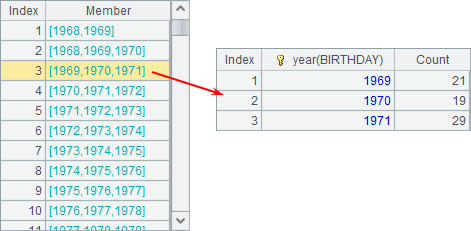A 1 =demo.query("select * from EMPLOYEE") 2 =A1.groups(DEPT;count(~):Count,sum(SALARY):TotalSalary) 3 =A1.groups(DEPT;count(GENDER=="M"):Male, count(GENDER=="F"):Female)

A2A3中直接根据DEPT字段分组汇总，注意写法和前面的例子略有不同，"~.”可以省略，结果和先分组再汇总时是相同的：A 1 =demo.query("select * from EMPLOYEE") 2 =A1.groups(DEPT;top(3,age(BIRTHDAY)))

A2中分组统计出每个部门中，最年轻的3个人的年龄：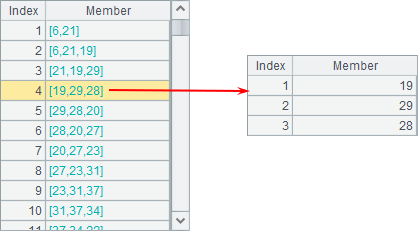A 1 =demo.query("select * from EMPLOYEE") 2 =A1.groups(DEPT;top(3,BIRTHDAY)) 3 =A2.new(DEPT,#2.(age(~))) 4 =A1.groups(DEPT;top(-3,age(BIRTHDAY)))

A2中先统计出每个部门中最小的3个生日，再在A3中进一步计算出年龄。A2A3中结果如下：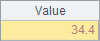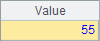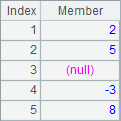A4A3中计算出的数据是相同的。